Now, i gram of hydrogen combines with 8 grams of oxygen in water ; and 8 is therefore chosen as the equivalent of oxygen ; for the definition of an equivalent is that amount of an element which will combine with or replace one part by weight of hydrogen. In ferrous oxide, since 22.22 grams of oxygen combine with 77.77 grams of iron, 8 grams of oxygen will combine with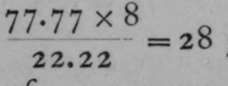grams of iron; and in ferric oxide, 8 grams ot oxygen are in combination with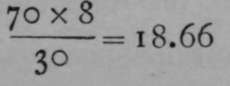grams of iron. Thus the equivalent of iron in ferrous oxide is 28, and in ferric oxide 18.66. The question now arises, Wha: is the atomic weight of iron ? We have seen that the specific heat of iron is 0.1138; and we know that the specific heat of solid hydrogen is probably 6. And as the specific heats of elements are inversely as their atomic weights, we have the proportion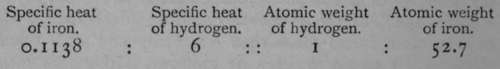.

The number 52.7 is nearly 2 x 28, and nearly 3 x 18.66 ; these products give 56 ; and it must be remembered that Dulong and Petit's law is not absolute, but merely an approximation ; hence 56 is accepted as the true atomic weight of iron.

The element sulphur forms a compound with hydrogen which has the following composition :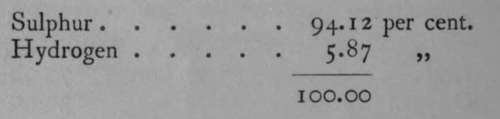.

The gas is 17.1 times as heavy as hydrogen; and this means that a molecule of hydrogen sulphide is 17.1 times as heavy as a molecule of hydrogen. But a molecule of hydrogen is believed to consist of two atoms ; hence a molecule of hydrogen sulphide is 34.2 times as heavy as an atom of hydrogen. Now, if this gas consists of one atom of hydrogen in combination with one atom of sulphur, then the atomic weight of sulphur will be the same as its equivalent, viz.,; but if it contain two atoms of hydrogen, then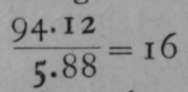the atomic weight of sulphur will be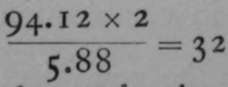. The first hypothesis is impossible, for then the molecular weight of hydrogen sulphide would be 16+1 = 17 ; whereas, it has been found to equal 34.2 ; but if two atoms of hydrogen are present in sulphide of hydrogen, the molecular weight is 32 4- 2 = 34 ; and this is nearly the same as the number found, viz., 34.2. It is, however, still possible that hydrogen sulphide consists of two atoms of hydrogen in union with two atoms of sulphur, in which case the atomic weight of sulphur might still be 16 ; but many other gaseous or gasifiable compounds of sulphur are known, and in none of them is the molecular weight such that they could be supposed to contain less than 32 parts of sulphur for each part of hydrogen, or its equivalent of another element. It is consequently regarded as improbable that the atomic weight of sulphur is less than 32 ; and that 32 is the correct number follows also from the determination of its specific heat.